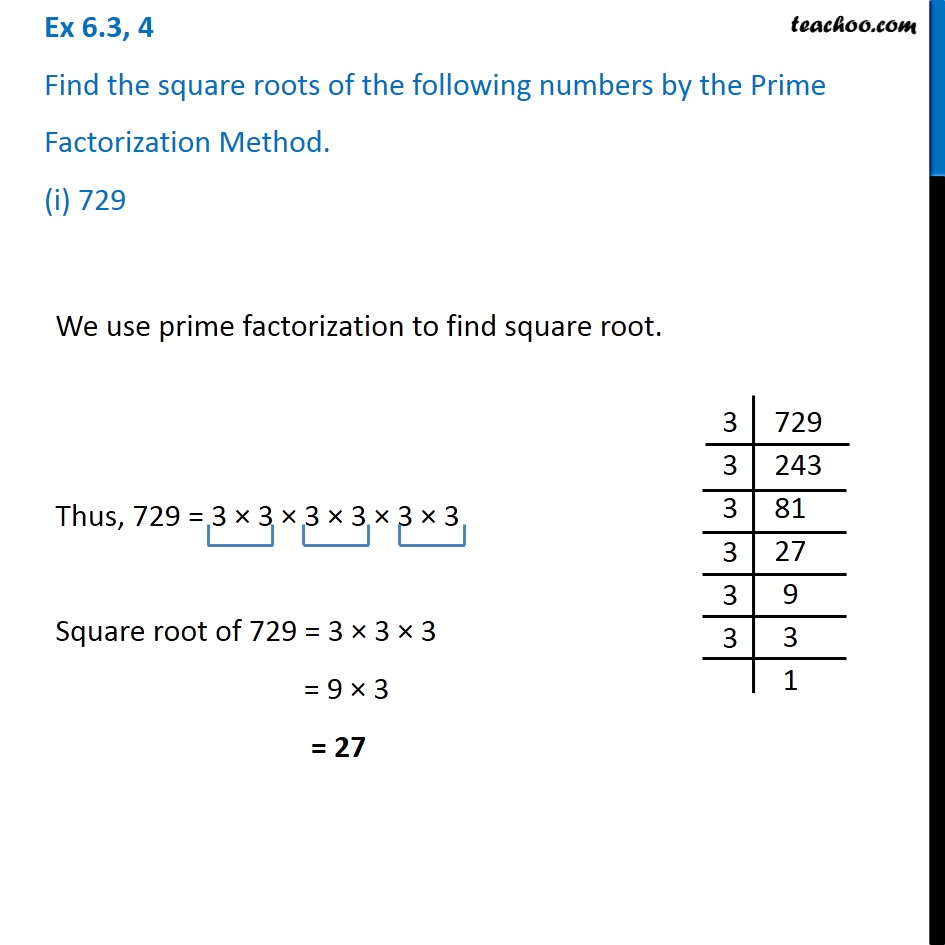1. Chapter 6 Class 8 Squares and Square Roots
2. Concept wise
3. Finding Square root through prime factorisation

Transcript

Ex 6.3, 4 Find the square roots of the following numbers by the Prime Factorization Method. (i) 729We use prime factorization to find square root. Thus, 729 = 3 × 3 × 3 × 3 × 3 × 3 Square root of 729 = 3 × 3 × 3 = 9 × 3 = 27